Courses

# Solved Objective Problems - Chemical Equilibrium, Class 11, Chemistry | EduRev Notes

## Class 11 : Solved Objective Problems - Chemical Equilibrium, Class 11, Chemistry | EduRev Notes

The document Solved Objective Problems - Chemical Equilibrium, Class 11, Chemistry | EduRev Notes is a part of the Class 11 Course Chemistry for JEE.
All you need of Class 11 at this link: Class 11

Problem 1 : The enthalpies of two reactions are DHand DH2 both positive with DH> DH1 . If the temperature of reacting system is increased from T1 to T2 predict which of the follow ing alternative is correct ?

(A)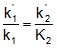(B)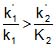(C)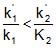(D)Ans. D Solution: As the temperature of reacting system is increased the equilibrium constant of reaction is also increased for endothermic reactions so for two reactions on increasing the temperature by equal amounts.

Problem 2: At a certain teperature 2 moles of crbonmonoxide and 3 moles of chlo rine were allowed to reach equilibrium according to the reaction CO Cl2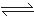COClin a 5 lit vessel. A t equilibrium if one mole of CO is present then equilibrium constant for the reaction is :

(A) 2 (B) 2. 5 (C) 3.0 (D) 4

Ans. B Solution: CO + Cl2COCl2

At t = 0 2 3 0

At equilbrium (2-1 ) (3-1 ) 1

Concentrations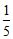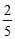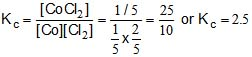Problem 3 : The numerical value of the equilibrium constant or any chemical change is affected by changing the

(A) concentration of product (B) catalyst

(C) concentration of reacting substance (D) temperature

Ans. D Solution : The equilibrium constant is unaffected by changing the concentration of products, catalyst and conentration of reactants. It is affected by changing the temperature.

Problem 4: The reaction : 3O2O3, DH= 69 , 000 calories is aided by :

(A) high temperature and low pressure (B) high temperature and high pressure

(C) low temperature and high pressure (D) low temperature and low pressure

Ans. B Solution : According to Le Chatalier principle formation of ozone is favoured by high temperature (endothermic reaction ) and high pressure.

Problem5 : In the homogenous reaction 2X2  + 2Y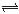2X2Y2, DH is negative for forward direction.The mass of X2Y2 increased at equilibrium by

(A) Raising the temperature (B) employing a suitable catalyst

(C) reducing the total pressure (D) raising the total pressure

Ans. D Solution: If the temperature is decreased and pressure is increased then reactions proceeds in for ward reaction.

Problem6: The equilibrium constant at 323oC is 1000. What would be its value in the presence of a catalyst in the forward reaction.

A + BC +  D 38 kcal

(A) 1000 x concentration of catalyst (B) 1000

(C)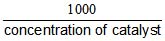(D) impossible to tell

Ans. B  Solution: The equilibrium constant vary only with temperature. At constant temperature it will not vary.

Problem 7 : Kp for the reaction : CO2(g) + H2(g)CO(g) + H2O(g) is found to be 160 at a given temperature. Originally equal number of moles of H2 and CO2 were placed in the flask . At equilibrium, the pressure of H2 is 1.20 atm. What is the partial pressure of CO and H2O?

(A) 4.80 atm each (B) 9.60 atm each

(C) 2.40 atm each (D) 1.20 atm each

Ans. A Solution: CO2  +HCO + H2O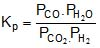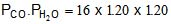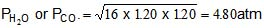Problem 8: Kc for the reaction : N2(g)+ O2(g)2NO(g) is 4.0 x 10-6 at 400 K.Kp for the above reaction is

(A) 2.4 x 10-3
(B) 4.0 x 10-6

(C) 4 x 10-6 x (RT)22
(D) None of these

Ans. B Solution: 2  O2 2NO

for this reaction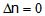Since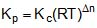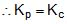Problem-9: Which one of the following reactions at equlibrium , with all reactans and products in the gaseous phase, would be uneffected by an increase in pressure.

(A) N2  +3H22NH3
(B) 2CO+ O22CO2

(C) 2H2 + O22H2O
(D) N2 + O22NO

Ans. D Solution: N O22NO, the number of molecules of reactants and products are the same so it is not affected by the pressure.

Problem 10: For the gas phase reaction 2NO(g)N2(g) O2(g) , DH = -43.5 kcal,which one of the following is true for N2(g) O2 (g) 2NO(g)

(A) K is independent of T
(B) K decreases as T decreases

(C) k increases as T decreases
(D) K varies with addition of NO

Ans. B

Solution: The given reaction N2(g) O2(g)2NO(g) is endothermic.Therefore, according to  Le-Chaltlier's Principle , high temperature favours forward reaction and hence K ncrease as T increase or K decrease as T decreases.

Offer running on EduRev: Apply code STAYHOME200 to get INR 200 off on our premium plan EduRev Infinity!

## Chemistry for JEE

223 videos|452 docs|334 tests

,

,

,

,

,

,

,

,

,

,

,

,

,

,

,

,

,

,

,

,

,

,

,

,

,

,

,

;